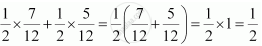Share

# An Urn Contains 5 Red and 5 Black Balls. a Ball is Drawn at Random, Its Colour is Noted and is Returned to the Urn. Moreover, 2 Additional Balls of the Colour Drawn Are Put in the Urn and Then a Ball is Drawn at Random. What is the Probability that the Second Ball is Red? - CBSE (Commerce) Class 12 - Mathematics

#### Question

An urn contains 5 red and 5 black balls. A ball is drawn at random, its colour is noted and is returned to the urn. Moreover, 2 additional balls of the colour drawn are put in the urn and then a ball is drawn at random. What is the probability that the second ball is red?

#### Solution

The urn contains 5 red and 5 black balls.

Let a red ball be drawn in the first attempt.

∴P (drawing a red ball) 5/10 = 1/2

If two red balls are added to the urn, then the urn contains 7 red and 5 black balls.

P (drawing a red ball) =7/12

Let a black ball be drawn in the first attempt.

∴ P (drawing a black ball in the first attempt) 5/10 = 1/2

If two black balls are added to the urn, then the urn contains 5 red and 7 black balls.

P (drawing a red ball) = 5/12

Therefore, probability of drawing second ball as red is`

Is there an error in this question or solution?

#### APPEARS IN

Solution An Urn Contains 5 Red and 5 Black Balls. a Ball is Drawn at Random, Its Colour is Noted and is Returned to the Urn. Moreover, 2 Additional Balls of the Colour Drawn Are Put in the Urn and Then a Ball is Drawn at Random. What is the Probability that the Second Ball is Red? Concept: Baye'S Theorem.
S